# Selina Concise Physics Class 10 ICSE Solutions Sound

## Selina Concise Physics Class 10 ICSE Solutions Sound

APlusTopper.com provides step by step solutions for Selina Concise ICSE Solutions for Class 10 Physics Chapter 7 Sound. You can download the Selina Concise Physics ICSE Solutions for Class 10 with Free PDF download option. Selina Publishers Concise Physics for Class 10 ICSE Solutions all questions are solved and explained by expert teachers as per ICSE board guidelines.

Selina ICSE Solutions for Class 10 Physics Chapter 7 Sound

Exercise 7(A)

Solution 2.

(a) Amplitude: The maximum displacement of the particle of medium on either side of its mean position is called the amplitude of wave. Its S.I. unit is metre (m).
(b) Frequency: The number of vibrations made by a particle of the medium in one second is called the frequency of the waves.
It is also defined as the number of waves passing through a point in one second. Its S.I. unit is hertz (Hz).
(c) Wavelength: The distance travelled by the wave in one time period of vibration of particle of medium is called its wavelength. Its S.I. unit is metre (m).
(d) Wave velocity: The distance travelled by a wave in one second is called its wave velocity. Its S.I. unit is metre per second (ms-1).

Solution 3.

(i) Wavelength (or speed) of the wave changes, when it passes from one medium to another medium.
(ii) Frequency of a wave does not change when it passes from one medium to another medium.

Solution 4.

Two factors on which the speed of a wave travelling in a medium depends are:

1. Density: The speed of sound is inversely proportional to the square root of density of the gas.
2. Temperature: The speed of sound increases with the increase in temperature.

Solution 5.

The light waves can travel in vacuum while sound waves need a material medium for propagation.
The light waves are electromagnetic waves while sound waves are the mechanical waves.

Solution 6.

If a person stands at some distance from a wall or a hillside and produces a sharp sound, he hears two distinct sounds: one is original sound heard almost instantaneously and the other one is heard after reflection from the wall or hillside, which is called echo.

The condition for the echo: An echo is heard only if the distance between the person producing the sound and the rigid obstacle is long enough to allow the reflected sound to reach the person at least 0.1 second after the original sound is heard.

Solution 7.

t = 2d/V = 2 x 12/340 = 24/340 < 0.1 seconds so the man will not be able to hear the echo. This is because the sensation of sound persists in our ears for about 0.1 second after the exciting stimulus ceases to act.

Solution 8.

The applications of echo:

1. Dolphins detect their enemy and obstacles by emitting the ultrasonic waves and hearing their echo.
2. In medical science, the echo method of ultrasonic waves is used for imaging the human organs such as the liver, gall bladder, uterus, womb etc. This is called ultrasonography.

Solution 9.

Sound is produced from a place at a known distance say, d at least 50 m from the reflecting surface. The time interval t in which the echo reaches the place from where the sound was produced, is noted by a stop watch having the least count 0.01 s. then the speed of sound is calculated by using the following relationSolution 10.

Bats, dolphin and fisherman detect their enemies or obstacles or position of fish by emitting/sending the ultrasonic waves and hearing/detecting the echo.

Solution 11.

Bats can produce and detect the sound of very high frequency up to about 1000kHz. The sounds produced by flying bats get reflected back from any obstacle in front of it. By hearing the echoes, bats come to know even in the dark where the obstacles are. So they can fly safely without colliding with the obstacles.

Solution 12.

The process of detecting obstacles with the help of echo is called sound ranging. It’s used by the animals like bats, dolphin to detect their enemies.

Solution 13.

The ultrasonic waves are used for the sound ranging. Ultrasonic waves have a frequency more than 20,000 Hz but the range of audibility of human ear is 20Hz to 20,000 Hz

Solution 14.

Sonar is sound navigation and ranging. Ultrasonic waves are sent in all directions from the ship and they are received on their return after reflection from the obstacles. They use the method of echo.

Solution 15.

In medical science, echo method of ultrasonic waves is used for the imaging of human organs such as liver, gall bladder, uterus, womb; which is called ultrasonography.

Solution 1 (MCQ).

17 m.

Explanation: An echo is heard distinctly if it reaches the ear at least 0.1 s after the original sound.
If d is the distance between the observer and the obstacle and V is the speed of sound, then the total distance travelled by the sound to reach the obstacle and then to come back is 2d and the time taken is,
t = Total distance travelled/Speed of sound = 2d/V
or, d = V t/2
Putting t = 0.1 s and V = 340 m/s in air at ordinary temperature, we get:
d = (340 x 0.1)/2 = 17 m
Thus, to hear an echo distinctly, the minimum distance between the source and the reflector in air is 17 m.

Solution 2 (MCQ).

Ultrasonic waves

Numericals

Solution 1.

(i) Frequency or the number of waves produced per second
= Velocity/Wavelength
= 24 / 20 x 10-2
= 120
(ii) Time = 1/ frequency = 1/ 120= 8.3 x 10-3 seconds

Solution 2.

Velocity = 2D/Time
350 = 2 x D/ 0.1
D = 350 x 0.1 / 2 = 17.5 m

Solution 3.

Velocity = 2D/Time
1400 = 2 x D/ 0.1
D = 1400 x 0.1/ 2 = 70 m

Solution 4.

(a) Velocity = 2D/Time
Time = 2 x 25 / 350 = 0.143 seconds
(b) Yes, because the reflected sound reaches the man 0.1 second after the original sound is heard and the original sound persists only for 0.1 second.

Solution 5.

Velocity = 2D/Time
3 x 108 = 2 x 45 x 1000/ Time
Time = 90000/ 3 x 10= 3 x 10-4 second

Solution 6.

Velocity = 2 x D/Time
Time after which an echo is heard = 2 D/Velocity = 2 x 48 / 320 = 0.3 seconds

Solution 7.

2 D = velocity x time
D = (velocity x time) / 2 = 1450 x 4 / 2 = 2900 m = 2.9 km

Solution 8.

5 vibrations by pendulum in 1 sec
So 8 vibrations in 8/5 seconds = 1.6 sec
Velocity = 2 x D/ time
340 = 2 x D/ 1.6
D = 340 x 1.6 / 2 = 272 m

Solution 9.

The distance of first cliff from the person, 2 x D1 = velocity x time
D1 = 320 x 4 / 2 = 640 m
Distance of the second cliff from the person, D2 = 320 x 6 / 2 = 960 m
Distance between cliffs = D1 + D2 = 640 + 960 = 1600 m

Solution 10.

Distance of hill from the man
D1 = velocity x time/ 2 =v x 5 / 2—————– (eqn 1)
Now, D1 – 310 = v x 3 / 2————————— (eqn 2)
By subtracting eqn 2 form eqn 1 ,we get310 = v x (5/2-3/2)
So, v = 310m/s

Solution 11.

Depth of the sea = velocity x time/2 = 1400 x 1.5 / 2 = 1050 m

Exercise 7(B)

Solution 1.

The vibrations of a body in the absence of any external force on it are called the free vibrations. Eg.: When we strike the keys of a piano, various strings are set into vibration at their natural frequencies.

Solution 2.

When each body capable of vibrating is set to vibrate freely and it vibrates with a frequency f. It is the natural frequency of vibration of the body.
The natural frequency of vibration of a body depends on the shape and size of the body.

Solution 3.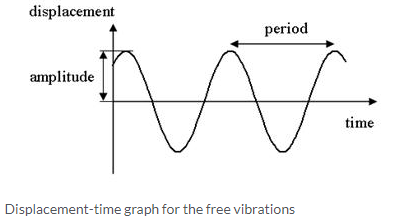Solution 4.

The free vibrations of a body occur only in vacuum because the presence of medium offer some resistance due to which the amplitude of the vibration does not remain constant, but it continuously decreases.

Solution 5.

The frequency of sound emitted due to vibration in an air column depends on the length of the air column.

Solution 6.

The frequency of the note produced in the air column can be increased by decreasing the length of the air column.

Solution 7.

The frequency of vibration of the stretched string can be increased by increasing the tension in the string, by decreasing the length of the string.

Solution 8.

A stringed instrument is provided with the provision for adjusting the tension of the string. By varying the tension, we can get the desired frequency.

Solution 9.

a) (i) Diagram is showing the principal note.
b) (iii)Diagram has frequency four times that of the first.
d) Ratio is 1:2

Solution 10.

Strings of different thickness are provided on a stringed instrument to produce different frequency sound waves because the natural frequency of vibration of a stretched string is inversely proportional to the radius (thickness) of the string.

Solution 11.

The frequency of vibrations of the blade can be lowered by increasing the length of the blade or by sticking a small weight on the blade at its free end.

Solution 12.

The presence of the medium offers some resistance to motion, so the vibrating body continuously loses energy due to which the amplitude of the vibration continuously decreases.

Solution 13.

The periodic vibrations of a body of decreasing amplitude in the presence of resistive force are called the damped vibrations.
The amplitude of the free vibrations remains constant and vibrations continue forever. But, the amplitude of damped vibrations decreases with time and ultimately the vibrations ceases.
For eg, When a slim branch of a tree is pulled and then released, it makes damped vibrations.
A tuning fork vibrating in air excute damped vibrations.

Solution 14.

1. Damped vibrations
2. Example: When a slim branch of a tree is pulled and then released, it makes damped vibrations.
3. The amplitude of vibrations gradually decreases due to the frictional (or resistive) force which the surrounding medium exerts on the body vibrating in it. As a result, the vibrating body continuously loses energy in doing work against the force of friction causing a decrease in its amplitude.
4. After sometime, the vibrating body loses all of its energy and stops vibrating.

Solution 15.

The tuning fork vibrates with the damped oscillations.

Solution 16.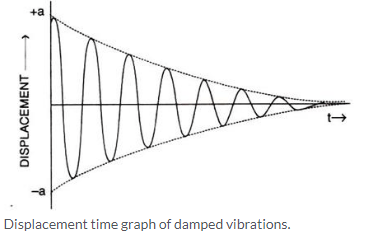Solution 17.

The vibrations of a body which take place under the influence of an external periodic force acting on it, are called the forced vibrations. For example: when guitar is played, the artist forces the strings of the guitar to execute forced vibrations.

Solution 18.

The vibrations of a body in the absence of any resistive force are called the free vibrations. The vibrations of a body in the presence of an external force are called forced vibrations.

In free vibrations, the frequency of vibration depends on the shape and size of the body. In forced vibrations, the frequency is equal to the frequency of the force applied.

Solution 19.

Resonance is a special case of forced vibrations. When the frequency of an externally applied periodic force on a body is equal to its natural frequency, the body rapidly begins to vibrate with an increased amplitude. This phenomenon is known as resonance.
Mount two identical tuning forks A and B of same frequency upon two separate sound boxes such that their open ends face each other as shown.If the prong A is struck on a rubber pad, it starts vibrating. On putting A on its sound box, tuning fork B also starts vibrating and a loud sound is heard. The vibrations produced in B are due to resonance.

Solution 20.

Condition for resonance:
Resonance occurs when the frequency of the applied force is exactly equal to the natural frequency of the vibrating body.

Solution 21.

forced,equal to the

Solution 22.

Solution 23.

At resonance, the body vibrates with large amplitude thus conveying more energy to the ears so a loud sound is heard.

Solution 24.

a) The vibrating tuning fork A produces the forced vibrations in the air column of its sound box. These vibrations are of large amplitude because of the large surface area of air in the sound box. They are communicated to the sound box of the fork B. The air column of B starts vibrating with the frequency of the fork A. Since the frequency of these vibrations is same as the natural frequency of the fork B, the fork B picks up these vibrations and starts vibrating due to resonance.
b) On putting the tuning fork A to vibrate, the other tuning fork B will also start vibrating. The vibrations produced in the second tuning fork B are due to resonance.

Solution 25.

(a) Set the pendulum A into vibration by displacing it to one side, normal to its length. It is observed that pendulum D also starts vibrating initially with a small amplitude and ultimately it acquires the same amplitude as the pendulum A initially had. When the amplitude of the pendulum D becomes maximum, the amplitude of the pendulum A becomes minimum since the total energy is constant. After some time the amplitude of the pendulum D will decreases and amplitude of A increases. The exchange of energy takes place only between the pendulums A and D because their natural frequencies are same. The pendulums B and C also vibrate, but with very small amplitudes.
(b) The vibrations produced in pendulum A are communicated as forced vibrations to the other pendulums B, C and D through XY. The pendulums B and C remain in the state of forced vibrations, while the pendulum D comes in the state of resonance.

Solution 26.

The phenomenon responsible for producing a loud audible sound is named resonance. The vibrating tuning fork causes the forced vibrations in the air column. For a certain length of air column, a loud sound is heard. This happens when the frequency of the air column becomes equal to the frequency of the tuning fork.

Solution 27.

(a) No loud sound is heard with the tubes A and C, but a loud sound is heard with the tube B.
(b) Resonance occurs with the air column in tube B whereas no resonance occurs in the air column of tubes A and C. The frequency of vibrations of air column in tube B is same as the frequency of vibrations of air column in tube D because the length of the air column in tube D is 20-18 = 2cm and that in tube B is 20-14 = 6 cm (3 times). On the other hand, the frequency of vibrations of air column in tubes A and C is not equal to the frequency vibrations of air column in tube B.
(c) When the frequency of vibrations of air column is equal to the frequency of the vibrating tuning fork, resonance occurs.

Solution 28.

When a troop crosses a suspension bridge, the soldiers are asked to break steps. The reason is that when soldiers march in steps, all the separate periodic forces exerted by them are in same phase and therefore forced vibrations of a particular frequency are produced in the bridge. Now, if the natural frequency of the bridge happens to be equal to the frequency of the steps, the bridge will vibrate with large amplitude due to resonance and suspension bridge could crumble

Solution 29.

The sound box is constructed such that the column of the air inside it, has a natural frequency which is the same as that of the strings stretched on it, so that when the strings are made to vibrate, the air column inside the box is set into forced vibrations. Since the sound box has a large area, it sets a large volume of air into vibration, the frequency of which is same as that of the string. So, due to resonance a loud sound is produced.

Solution 30.

When we tune a radio receiver, we merely adjust the values of the electronic components to produce vibrations of frequency equal to that of the radio waves which we want to receive. When the two frequencies match, due to resonance the energy of the signal of that particular frequency is received from the incoming waves. The signal received is then amplified in the receiver set.
The phenomenon involved is resonance. It is a special case of forced vibrations. When the frequency of an externally applied periodic force on a body is equal to its natural frequency, the body rapidly begins to vibrate with an increased amplitude. This phenomenon is known as resonance.

Solution 1 (MCQ).

It executes free vibrations.
Hint: The periodic vibrations of a body of constant amplitude in the absence of any external force on it are called free vibrations.

Solution 2 (MCQ).

Forced vibrations
Hint: The vibrations of a body which take place under the influence of external periodic force acting on it are called the forced vibrations.

Solution 3 (MCQ).

A tuning fork of frequency 256 Hz will resonate with another tuning fork of frequency 256 Hz.
Hint: Resonance occurs when the frequency of an externally applied periodic force on the body is equal to its natural frequency.

Exercise 7(C)

Solution 1.

The following three characteristics of sound are:

1. Loudness
2. Pitch or shrillness
3. Quality or timber.

Solution 2.

(a) Amplitude – The louder sound corresponds to the wave of large amplitude.
(b) Loudness is directly proportional to the square of amplitude.

Solution 3.

Loudness will be four times because loudness is directly proportional to the square of amplitude.

Solution 4.

(a) Ratio of loudness will be 1:9
(b) The ratio of frequency will be 1:1

Solution 5.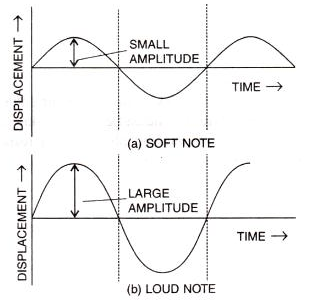Solution 6.

The unit of loudness is phon.

Solution 7.

Because the board provides comparatively a large area and forces a large volume of air to vibrate and thereby increases the sound energy reaching our ears.

Solution 8.

The intensity at any point of the medium is the amount of sound energy passing per second normally through unit area at that point. Its unit is microwatt per metre2.

Solution 9.

Relationship between loudness L and intensity I is given as:
L = K log I, where K is a constant of proportionality.

Solution 10.

The intensity at any point of the medium is the amount of sound energy passing per second normally through unit area at that point.
The loudness of a sound depends on the energy conveyed by the sound wave near the eardrum of the listener. Loudness, being a sensation, also depends on the sensitivity of the ears of the listener. Thus the loudness of sound of a given intensity may differ from listener to listener. Further, two sounds of the same intensity but of different frequencies may differ in loudness even to the same listener because of the sensitivity of ears is different for different frequencies. So, loudness is a subjective quantity while intensity being a measurable quantity is an objective quantity for the sound wave.

Solution 11.

The loudness of the sound heard depends on:

1. Loudness is proportional to the square of the amplitude.
2. Loudness is inversely proportional to the square of distance.
3. Loudness depends on the surface area of the vibrating body.

Solution 12.

Decibel is the unit used to measure the sound level

Solution 13.

Upto 120 dB

Solution 14.

The disturbance produced in the environment due to undesirable loud and harsh sound of level above 120 dB from the various sources such as loudspeaker, moving vehicles etc. is called noise pollution.

Solution 15.

Pitch of sound is determined by its wavelength or the frequency. Two notes of the same amplitude and sounded on the same instrument will differ in pitch when their vibrations are of different wavelengths or frequencies.

Solution 16.

Pitch

Solution 17.

Pitch is the characteristic of sound which enables us to distinguish different frequencies sound. Pitch is the characteristic of sound by which an acute note can be distinguished from a grave or flat note.

Solution 18.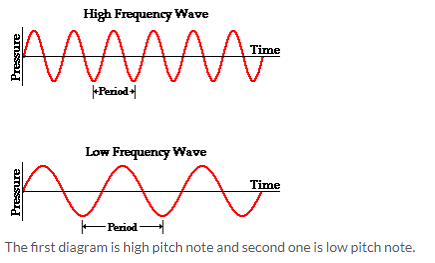Solution 19.

As the water level in a bottle kept under a water tap rises, the length of air column decreases, so the frequency of sound produced increases i.e., sound becomes shriller and shriller. Thus by hearing sound from a distance, one can get the idea of water level in the bottle.

Solution 20.

Trumpet. Because its frequency is highest.

Solution 21.

(a) increases
(b) one-fourth

Solution 23.

Quality or timber of sound.

Solution 24.

The two sounds of same loudness and same pitch produced by different instruments differ due to their different waveforms.
The waveforms depend on the number of the subsidiary notes and their relative amplitude along with the principal note.
Diagram below shows the wave patterns of two sounds of same loudness and same pitch but emitted by two different instruments. They produce different sensation to ears because they differ in waveforms: one is a sine wave, while the other is a triangular wave.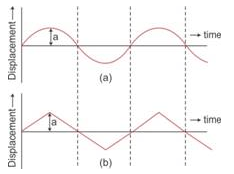Solution 25.

Since the guitars are identical, they will have a similar waveform and so the similar quality.

Solution 26.

Different instruments emit different subsidiary notes. A note played on one instrument has a large number of subsidiary notes while the same note when played on other instrument contains only few subsidiary notes. So they have different waveforms.

Solution 27.

It is because the vibrations produced by the vocal chord of each person have a characteristic waveform which is different for different persons.

Solution 28.

1. Frequency
2. Amplitude
3. Waveform

Solution 29.

1. Loudness
2. Quality or timbre
3. Pitch

Solution 30.Solution 31.

1. IV
2. I
3. II

Solution 33.

(i) b, since amplitude is largest
(ii) a, since frequency is lowest

Solution 34.

Musical note is pleasant, smooth and agreeable to the ear while noise is harsh, discordant and displeasing to the ear.
In musical note, waveform is regular while in noise waveform is irregular.

Solution 1 (MCQ).

By reducing the amplitude of the sound wave, its loudness decreases.
Hint: Loudness of sound is proportional to the square of the amplitude.

Solution 2 (MCQ).

Waveforms
Explanation: The waveform of a sound depends on the number of the subsidiary notes and their relative amplitude along with the principal note. The resultant vibration obtained by the superposition of all these vibrations gives the waveform of sound.

Solution 3 (MCQ).

B is shrill, A is grave
Explanation: Shrillness or pitch of a sound is directly proportional to the frequency of the sound wave. Greater the frequency, shriller will be the note.

More Resources for Selina Concise Class 10 ICSE Solutions